# Factoring polynomials

##By MathPlanetVideos

Factor 2x2-4xy+2y2=2(x2-2xy+y2)=2(x-y) 2# Matrix Scalar Multiplication - YouTube

##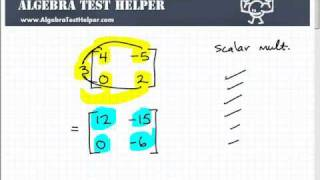By TabletClass Math

Youtube Presents Matrix Scalar Multiplication an educational video resources on algebra 1 resources# Matrix multiplication practice-Homework Help

##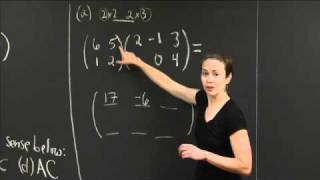By MIT OpenCourseWare

This is a series of video lecture tutorial on Multivariable Calculus and helps students step-by-step on solving homework problems related to Multivariable Calculus. . ....# How to Factor Polynomials by Grouping

##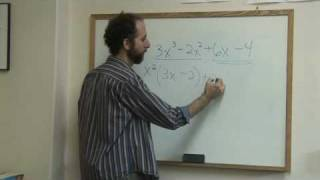By eHow

In math, factoring by grouping means that the greatest common factor needs to be found. In this video learn how to factor four different terms through grouping with help from a math author and teacher. As narrator explains there is text of what he says shown on the bottom of the screen.# Scalar Multiplication of a Matrix

##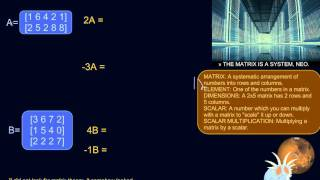By Mathceratops

Scalar Multiplication of a Matrix# Linear Algebra: Matrix Multiplication

##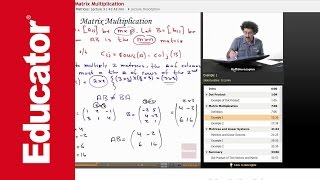By Educator

Linear Algebra: Matrix Multiplication# Scalar multiplication | Matrices | Precalculus | Khan Academy

##By Khan Academy

Scalar multiplication | Matrices | Precalculus | Khan Academy# (correction) scalar multiplication of row | Matrix transformations | Linear Algebra | Khan Academy

##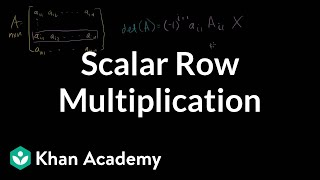By Khan Academy

Correction of last video showing that the determinant when one row is multiplied by a scalar is equal to the scalar times the determinant# Factoring Polynomials

##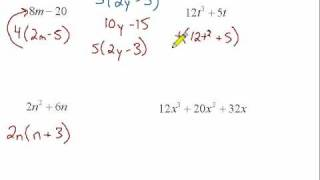By Marty Brandl

This video looks at factoring simple binomials and trinomials. It includes four examples.# Vector Addition and Scalar Multiplication, Example 1

##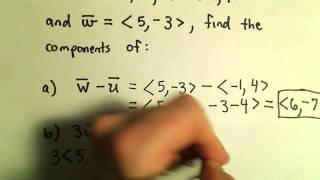By PatrickJMT

Vector Addition and Scalar Multiplication, Example 1. In this video, we look at vector addition and scalar multiplication algebraically using the component form of the vector. I do not graph the vectors in this video (but do in others).# Vector Addition and Scalar Multiplication, Example 2

##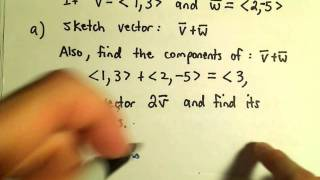By PatrickJMT

Vector Addition and Scalar Multiplication, Example 2. In this video I add two vectors in component form and also sketch the vectors to illustrate how to add vectors graphically (very useful stuff!).# Example 3: Factor a quartic binomial with two variables by taking a common factor | Khan Academy

##By Khan Academy

Khan Academy presents Factor polynomials using the GCF, an educational video resource on math.# More on matrix addition and scalar multiplication | Linear Algebra | Khan Academy

##By Khan Academy

More on Matrix Addition and Scalar Multiplication# More on matrix addition and scalar multiplication | Linear Algebra | Khan Academy

##By Khan Academy

More on Matrix Addition and Scalar Multiplication# Vector Addition and Scalar Multiplication, Example 2

##By PatrickJMT

Vector Addition and Scalar Multiplication, Example 2. In this video I add two vectors in component form and also sketch the vectors to illustrate how to add vectors graphically (very useful stuff!).# Multiplying and Dividing Decimals | 7.NS.A.2c | 7th Grade Math

##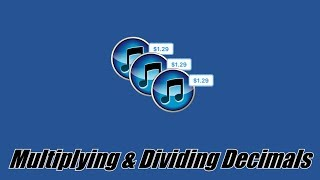By ParksMath | Todd Parks

ParksMath explains how to multiply and divide decimals numbers without the use of a calculator. Understanding how to change a decimal number so that it is an integer, makes multiplying and dividing decimals much easier. This quick tutorial will give you thee tools that you need to find the product or quotient of any decimal number.# Vectors : scalar multiplication, addition and subtraction : ExamSolutions

##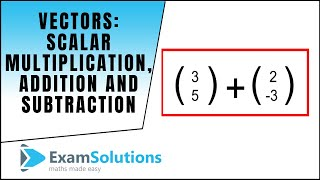By ExamSolutions

Youtube Presents Vectors : scalar multiplication, addition and subtraction : ExamSolutions an educational video resources on english language arts.# Math Antics - Multiplying Fractions

##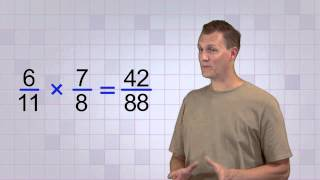By# Math Antics - Multi-Digit Multiplication Pt 2

##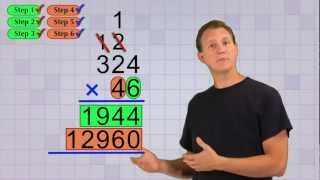By mathantics# Multiplying Fractions and Mixed Numbers with John and Aiden

##By Anywhere Math